# Selina Solutions Concise Mathematics Class 6 Chapter 27: Quadrilateral Exercise 27(A)

Selina Solutions Concise Mathematics Class 6 Chapter 27 Quadrilateral Exercise 27(A) are available in PDF format to boost the exam preparation of students. The method of finding the angles of a quadrilateral is the main concept talked about under this exercise. Multiple solved examples are present to help students grasp the various tricks of solving complex problems with ease. Students can find Selina Solutions Concise Mathematics Class 6 Chapter 27 Quadrilateral Exercise 27(A) PDF, from the links provided here.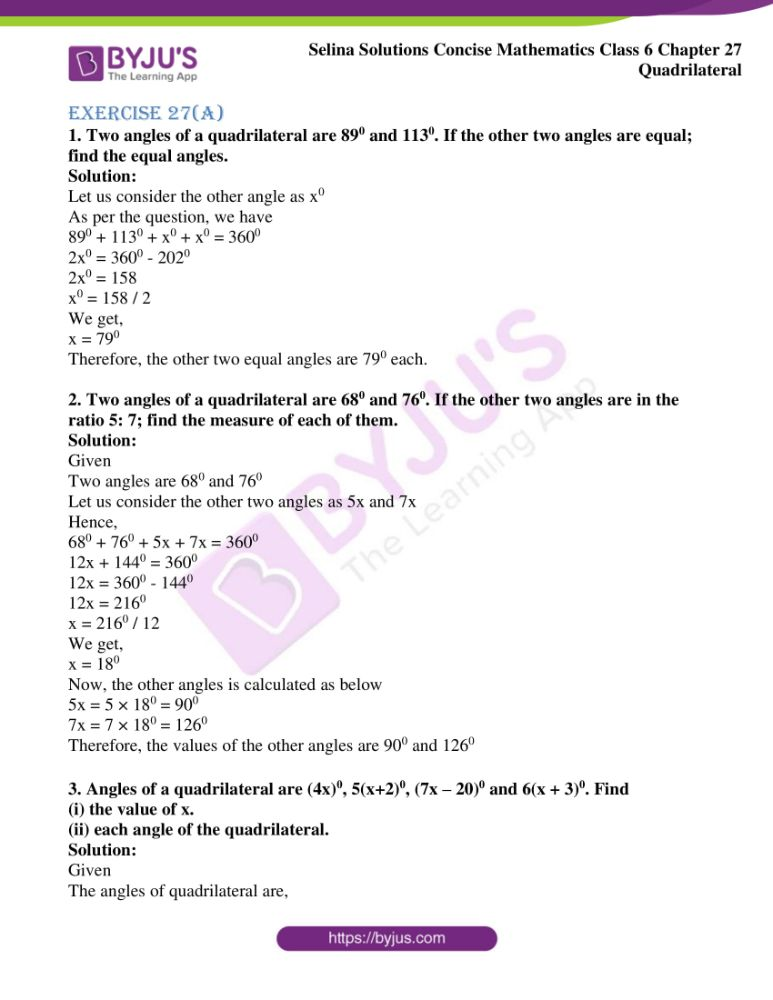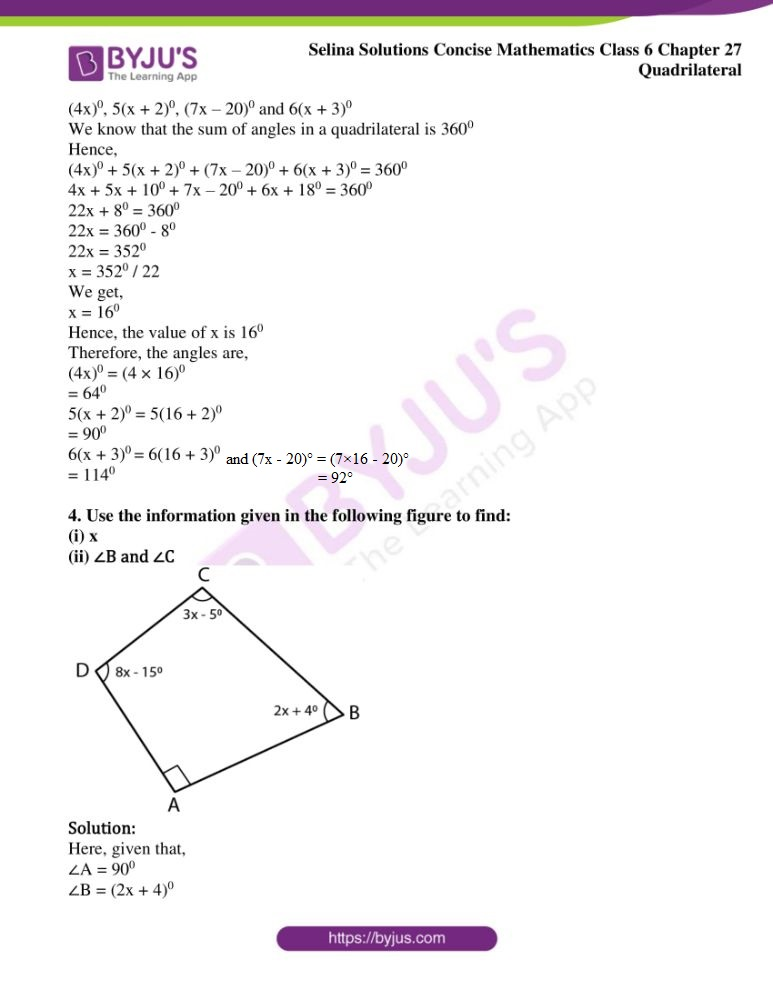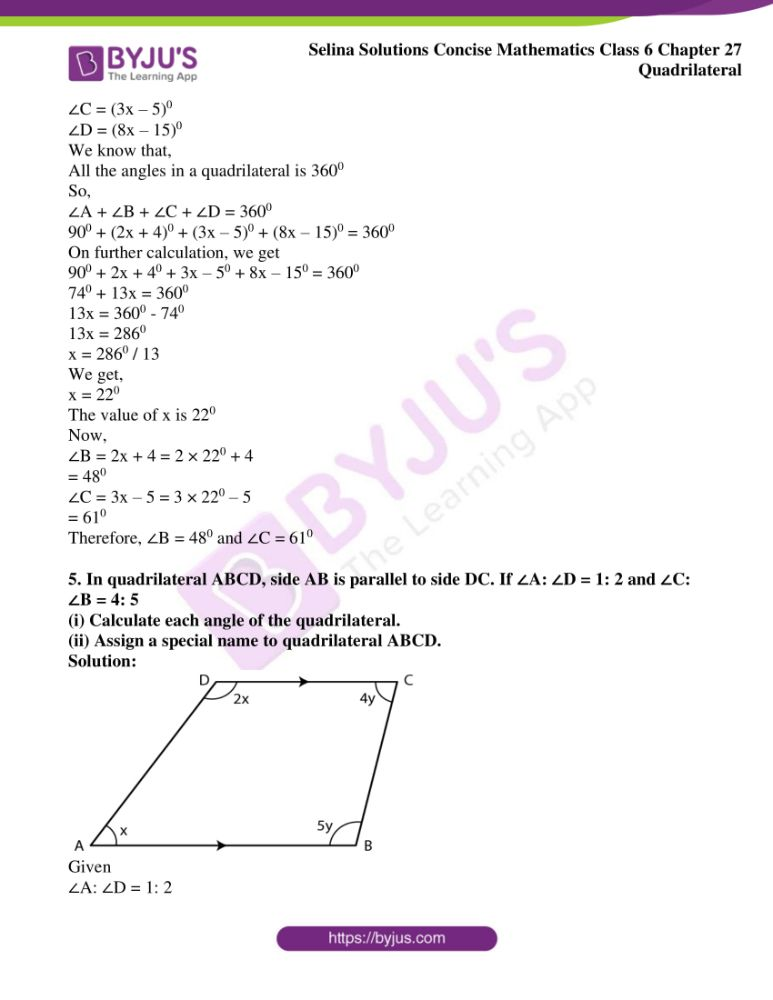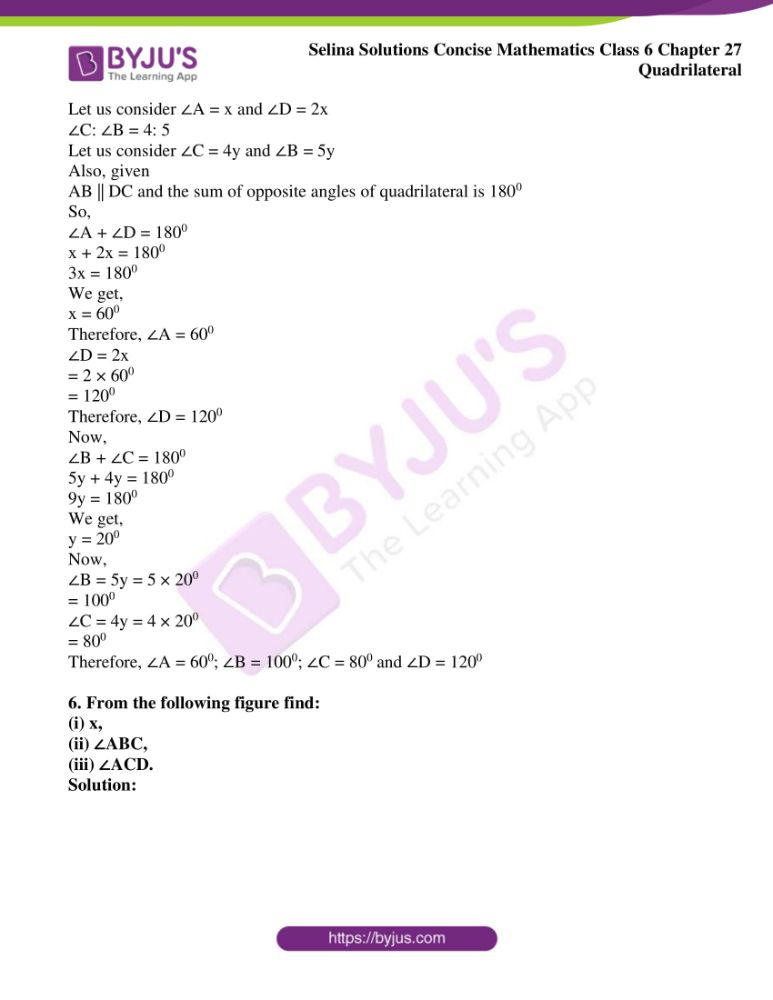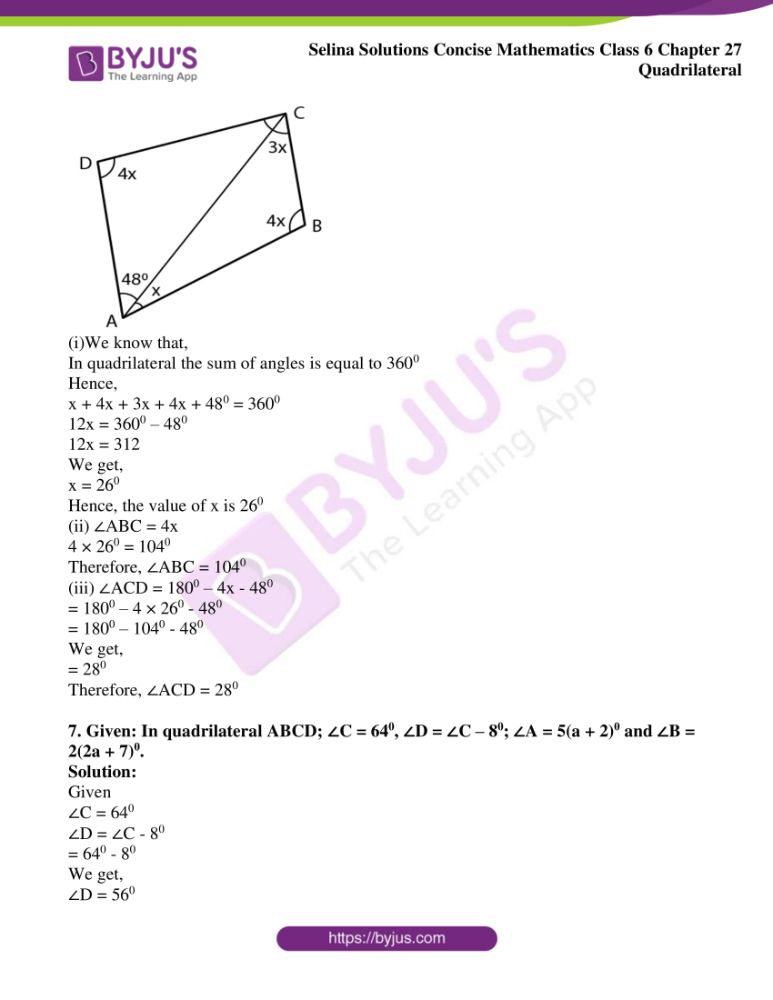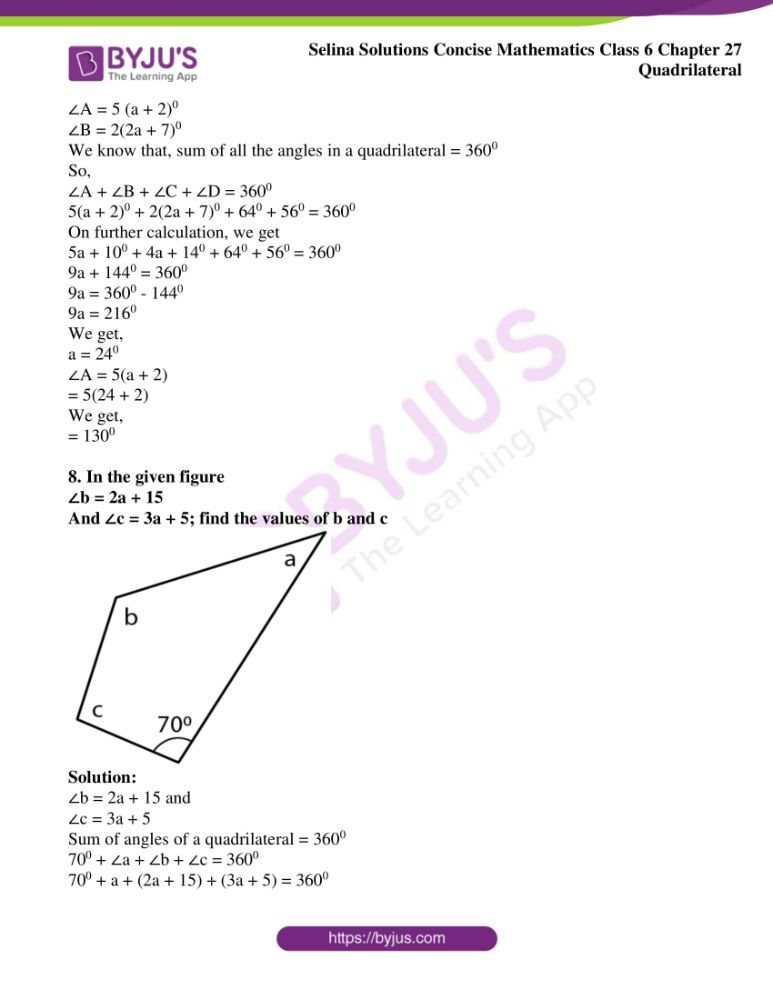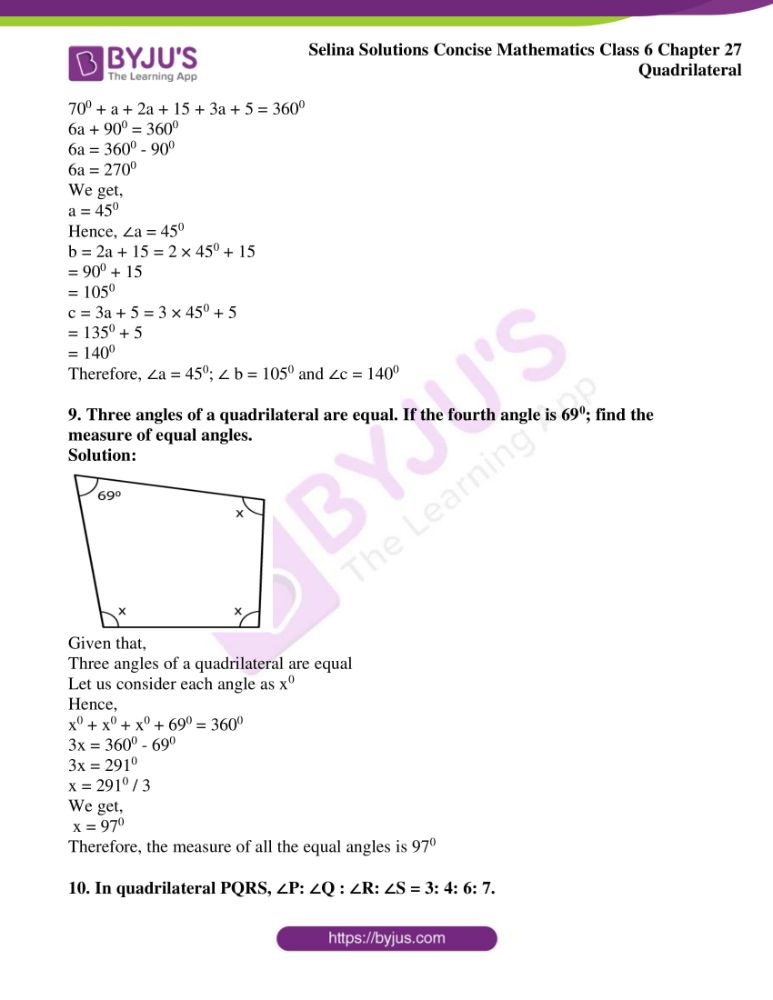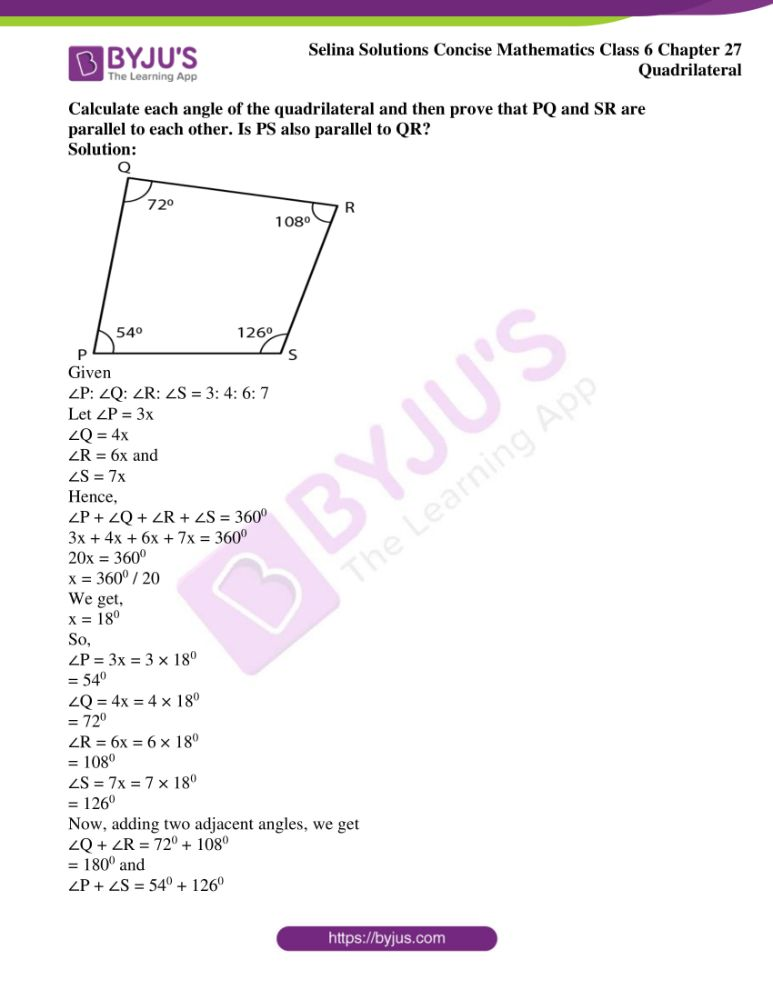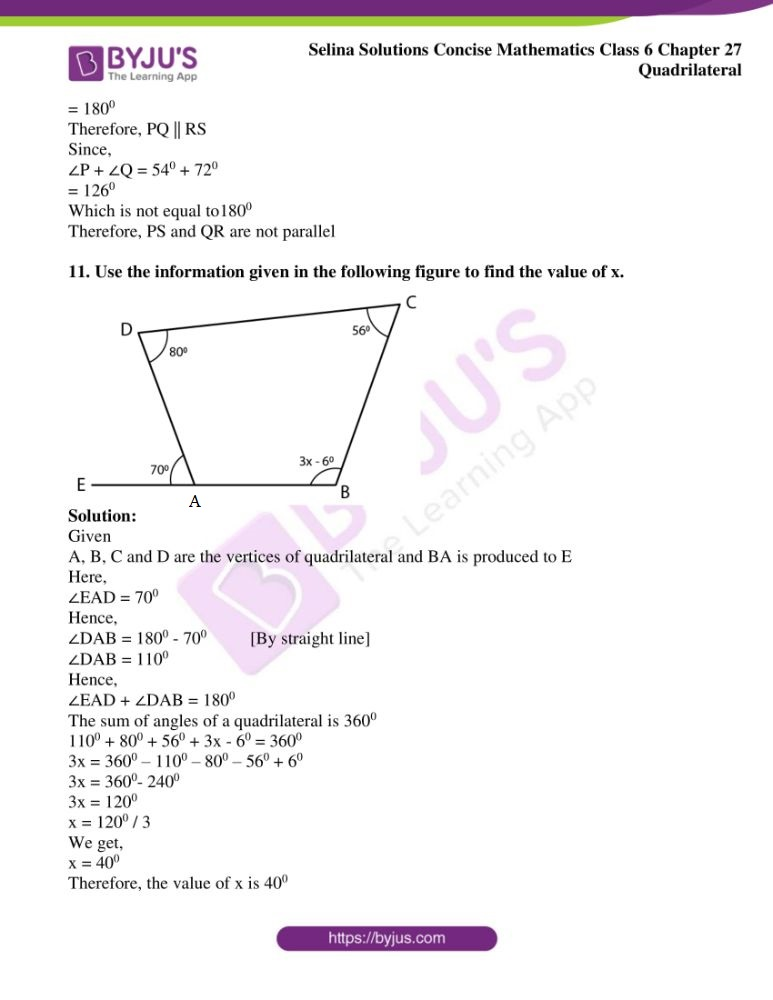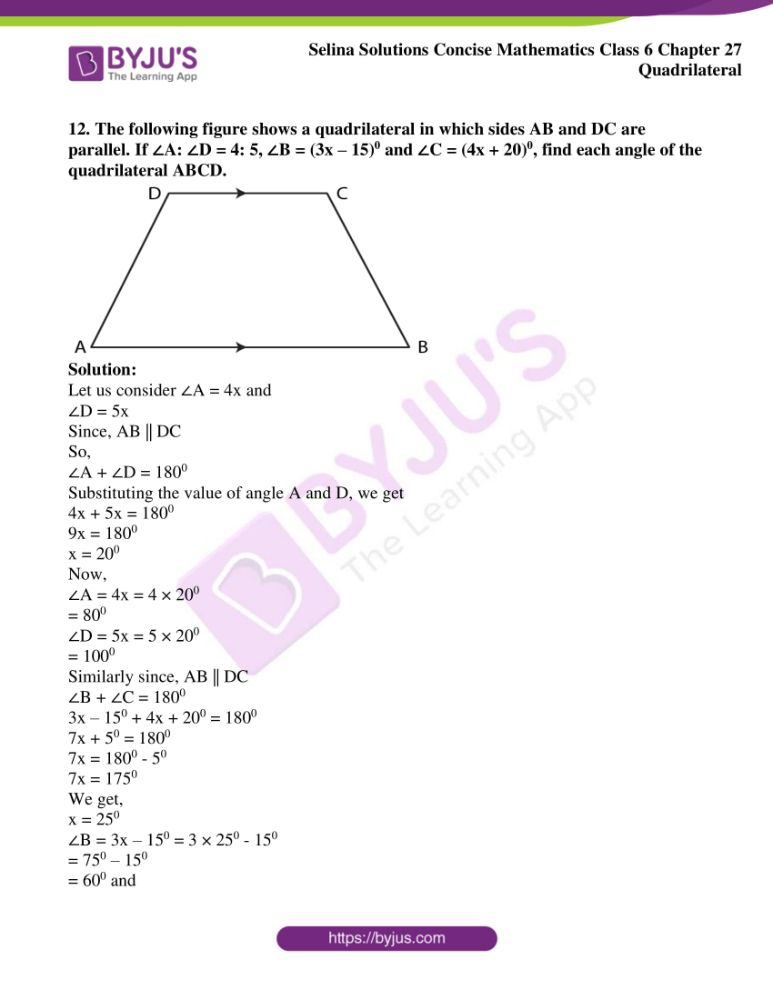### Access another exercise of Selina Solutions Concise Mathematics Class 6 Chapter 27: Quadrilateral

Exercise 27(B) Solutions

### Access Selina Solutions Concise Mathematics Class 6 Chapter 27 Quadrilateral Exercise 27(A)

Exercise 27(A)

1. Two angles of a quadrilateral are 890 and 1130. If the other two angles are equal; find the equal angles.

Solution:

Let us consider the other angle as x0

As per the question, we have

890 + 1130 + x0 + x0 = 3600

2x0 = 3600 – 2020

2x0 = 158

x0 = 158 / 2

We get,

x = 790

Therefore, the other two equal angles are 790 each.

2. Two angles of a quadrilateral are 680 and 760. If the other two angles are in the ratio 5: 7; find the measure of each of them.

Solution:

Given

Two angles are 680 and 760

Let us consider the other two angles as 5x and 7x

Hence,

680 + 760 + 5x + 7x = 3600

12x + 1440 = 3600

12x = 3600 – 1440

12x = 2160

x = 2160 / 12

We get,

x = 180

Now, the other angles is calculated as below

5x = 5 × 180 = 900

7x = 7 × 180 = 1260

Therefore, the values of the other angles are 900 and 1260

3. Angles of a quadrilateral are (4x)0, 5(x+2)0, (7x – 20)0 and 6(x + 3)0. Find

(i) the value of x.

(ii) each angle of the quadrilateral.

Solution:

Given

(4x)0, 5(x + 2)0, (7x – 20)0 and 6(x + 3)0

We know that the sum of angles in a quadrilateral is 3600

Hence,

(4x)0 + 5(x + 2)0 + (7x – 20)0 + 6(x + 3)0 = 3600

4x + 5x + 100 + 7x – 200 + 6x + 180 = 3600

22x + 80 = 3600

22x = 3600 – 80

22x = 3520

x = 3520 / 22

We get,

x = 160

Hence, the value of x is 160

Therefore, the angles are,

(4x)0 = (4 × 16)0

= 640

5(x + 2)0 = 5(16 + 2)0

= 900

6(x + 3)0 = 6(16 + 3)0

= 1140

And,

(7x – 20)° = (7×16 – 20)°

= 92°

4. Use the information given in the following figure to find:

(i) x

(ii) ∠B and ∠C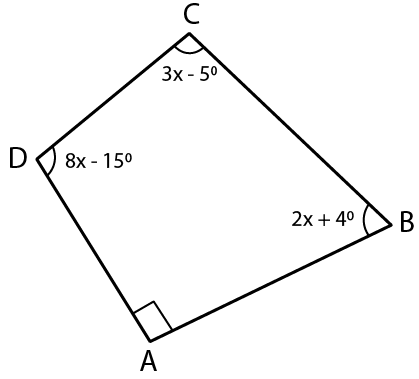Solution:

Here, given that,

∠A = 900

∠B = (2x + 4)0

∠C = (3x – 5)0

∠D = (8x – 15)0

We know that,

All the angles in a quadrilateral is 3600

So,

∠A + ∠B + ∠C + ∠D = 3600

900 + (2x + 4)0 + (3x – 5)0 + (8x – 15)0 = 3600

On further calculation, we get

900 + 2x + 40 + 3x – 50 + 8x – 150 = 3600

740 + 13x = 3600

13x = 3600 – 740

13x = 2860

x = 2860 / 13

We get,

x = 220

The value of x is 220

Now,

∠B = 2x + 4 = 2 × 220 + 4

= 480

∠C = 3x – 5 = 3 × 220 – 5

= 610

Therefore, ∠B = 480 and ∠C = 610

5. In quadrilateral ABCD, side AB is parallel to side DC. If ∠A: ∠D = 1: 2 and ∠C: ∠B = 4: 5

(i) Calculate each angle of the quadrilateral.

(ii) Assign a special name to quadrilateral ABCD.

Solution: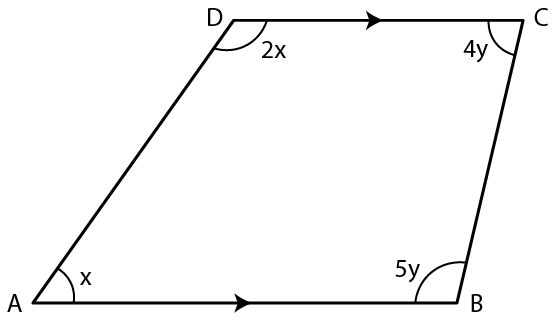Given

∠A: ∠D = 1: 2

Let us consider ∠A = x and ∠D = 2x

∠C: ∠B = 4: 5

Let us consider ∠C = 4y and ∠B = 5y

Also, given

AB || DC and the sum of opposite angles of quadrilateral is 1800

So,

∠A + ∠D = 1800

x + 2x = 1800

3x = 1800

We get,

x = 600

Therefore, ∠A = 600

∠D = 2x

= 2 × 600

= 1200

Therefore, ∠D = 1200

Now,

∠B + ∠C = 1800

5y + 4y = 1800

9y = 1800

We get,

y = 200

Now,

∠B = 5y = 5 × 200

= 1000

∠C = 4y = 4 × 200

= 800

Therefore, ∠A = 600; ∠B = 1000; ∠C = 800 and ∠D = 1200

6. From the following figure find:

(i) x,

(ii) ∠ABC,

(iii) ∠ACD.

Solution: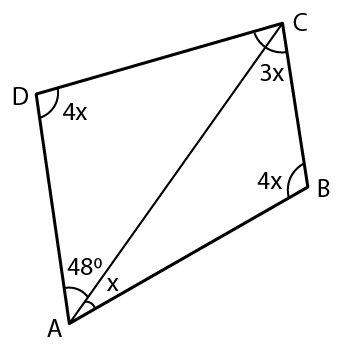(i)We know that,

In quadrilateral the sum of angles is equal to 3600

Hence,

x + 4x + 3x + 4x + 480 = 3600

12x = 3600 – 480

12x = 312

We get,

x = 260

Hence, the value of x is 260

(ii) ∠ABC = 4x

4 × 260 = 1040

Therefore, ∠ABC = 1040

(iii) ∠ACD = 1800 – 4x – 480

= 1800 – 4 × 260 – 480

= 1800 – 1040 – 480

We get,

= 280

Therefore, ∠ACD = 280

7. Given: In quadrilateral ABCD; ∠C = 640, ∠D = ∠C – 80; ∠A = 5(a + 2)0 and ∠B = 2(2a + 7)0.

Solution:

Given

∠C = 640

∠D = ∠C – 80

= 640 – 80

We get,

∠D = 560

∠A = 5 (a + 2)0

∠B = 2(2a + 7)0

We know that, sum of all the angles in a quadrilateral = 3600

So,

∠A + ∠B + ∠C + ∠D = 3600

5(a + 2)0 + 2(2a + 7)0 + 640 + 560 = 3600

On further calculation, we get

5a + 100 + 4a + 140 + 640 + 560 = 3600

9a + 1440 = 3600

9a = 3600 – 1440

9a = 2160

We get,

a = 240

∠A = 5(a + 2)

= 5(24 + 2)

We get,

= 1300

8. In the given figure

∠b = 2a + 15

And ∠c = 3a + 5; find the values of b and c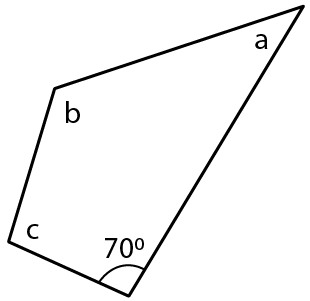Solution:

∠b = 2a + 15 and

∠c = 3a + 5

Sum of angles of a quadrilateral = 3600

700 + ∠a + ∠b + ∠c = 3600

700 + a + (2a + 15) + (3a + 5) = 3600

700 + a + 2a + 15 + 3a + 5 = 3600

6a + 900 = 3600

6a = 3600 – 900

6a = 2700

We get,

a = 450

Hence, ∠a = 450

b = 2a + 15 = 2 × 450 + 15

= 900 + 15

= 1050

c = 3a + 5 = 3 × 450 + 5

= 1350 + 5

= 1400

Therefore, ∠a = 450; ∠ b = 1050 and ∠c = 1400

9. Three angles of a quadrilateral are equal. If the fourth angle is 690; find the measure of equal angles.

Solution: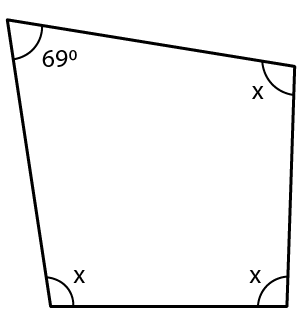Given that,

Three angles of a quadrilateral are equal

Let us consider each angle as x0

Hence,

x0 + x0 + x0 + 690 = 3600

3x = 3600 – 690

3x = 2910

x = 2910 / 3

We get,

x = 970

Therefore, the measure of all the equal angles is 970

10. In quadrilateral PQRS, ∠P: ∠Q : ∠R: ∠S = 3: 4: 6: 7.

Calculate each angle of the quadrilateral and then prove that PQ and SR are parallel to each other. Is PS also parallel to QR?

Solution: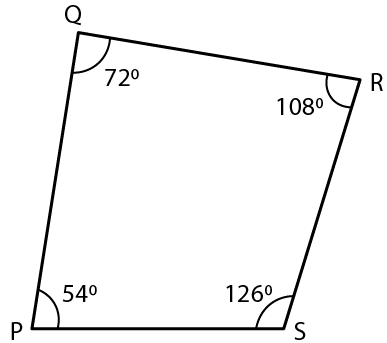Given

∠P: ∠Q: ∠R: ∠S = 3: 4: 6: 7

Let ∠P = 3x

∠Q = 4x

∠R = 6x and

∠S = 7x

Hence,

∠P + ∠Q + ∠R + ∠S = 3600

3x + 4x + 6x + 7x = 3600

20x = 3600

x = 3600 / 20

We get,

x = 180

So,

∠P = 3x = 3 × 180

= 540

∠Q = 4x = 4 × 180

= 720

∠R = 6x = 6 × 180

= 1080

∠S = 7x = 7 × 180

= 1260

∠Q + ∠R = 720 + 1080

= 1800 and

∠P + ∠S = 540 + 1260

= 1800

Therefore, PQ || RS

Since,

∠P + ∠Q = 540 + 720

= 1260

Which is not equal to1800

Therefore, PS and QR are not parallel

11. Use the information given in the following figure to find the value of x.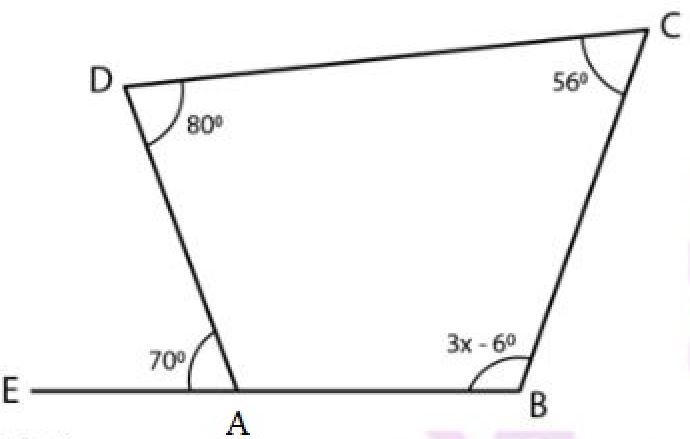Solution:

Given

A, B, C and D are the vertices of quadrilateral and BA is produced to E

Here,

Hence,

∠DAB = 1800 – 700 [By straight line]

∠DAB = 1100

Hence,

The sum of angles of a quadrilateral is 3600

1100 + 800 + 560 + 3x – 60 = 3600

3x = 3600 – 1100 – 800 – 560 + 60

3x = 3600– 2400

3x = 1200

x = 1200 / 3

We get,

x = 400

Therefore, the value of x is 400

12. The following figure shows a quadrilateral in which sides AB and DC are parallel. If ∠A: ∠D = 4: 5, ∠B = (3x – 15)0 and ∠C = (4x + 20)0, find each angle of the quadrilateral ABCD.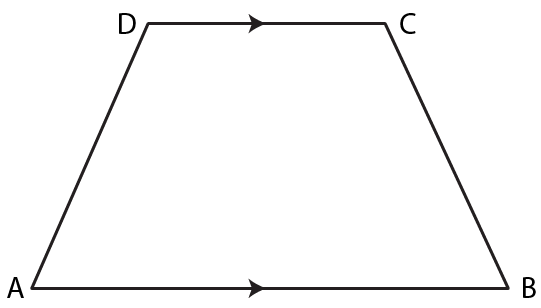Solution:

Let us consider ∠A = 4x and

∠D = 5x

Since, AB || DC

So,

∠A + ∠D = 1800

Substituting the value of angle A and D, we get

4x + 5x = 1800

9x = 1800

x = 200

Now,

∠A = 4x = 4 × 200

= 800

∠D = 5x = 5 × 200

= 1000

Similarly since, AB || DC

∠B + ∠C = 1800

3x – 150 + 4x + 200 = 1800

7x + 50 = 1800

7x = 1800 – 50

7x = 1750

We get,

x = 250

∠B = 3x – 150 = 3 × 250 – 150

= 750 – 150

= 600 and

∠C = 4x + 200 = 4 × 250 + 200

= 1000 + 200

= 1200